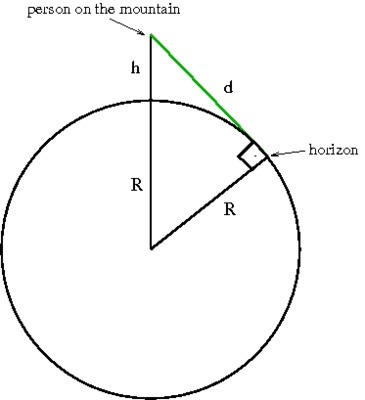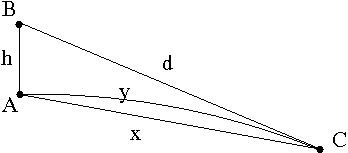# Neglecting the Curvature of the Earth

Alignments to Content Standards: G-C.A.2 G-SRT.C.8

Jerry and Ashley are trying to find out if it is possible to see the lowest point in the USA from the highest point in the USA. It turns out that the highest point in the United States, the peak of Mount Whitney, is only 85 miles from the lowest area, a large flat stretch of land in Death Valley called Badwater Basin.

Jerry thinks that the curvature of the earth will block the view of Badwater Basin from the peak of Mount Whitney. Ashley doesn't think that this is an issue and that they can neglect the curvature of the earth in their investigation.

As it turns out, it is not possible to see Badwater Basin from Mt. Whitney because the Panamint Range blocks the view. But what if there were no obstacles in the way? More precisely:

Standing on top of Mt. Whitney, is it possible to see a point on the surface of the earth 85 miles away, or would the curvature of the earth prevent this?

## IM Commentary

This task applies geometric concepts, namely properties of tangents to circles and of right triangles, in a modeling situation. The key geometric point in this task is to recognize that the line of sight from the mountain top towards the horizon is tangent to the earth. We can then use a right triangle where one leg is tangent to a circle and the other leg is the radius of the circle to investigate this situation.

As with any serious modeling task, there are several places where we need to make simplifying assumptions. We mention some of these explicitly here: First and foremost, we note the assumption that the earth is perfectly spherical in shape. This is a non-trivial but important simplifying assumption, as geometry on an "oblate ellipsoid" is vastly more complicated. Next, we note that we make use of the fact that Badwater Basin is a large expanse of land at an elevation (282 feet below sea level) negligible compared to that of Mount Whitney (14,505 feet above sea level), rather a single point deep in a ravine. This is what allows us to rephrase the question simply as the visibility of a point 85 miles away. A picture of Badwater Basin can be found here, and may be useful for the instructor and/or the students for visualizing the validity of this assumption.

Hand-in-hand with modeling assumptions are several numerical approximations being made in the solution, giving students ample opportunity to discuss what it means to "attend to precision" (SMP 6). There are several places in the provided solution where we are approximating distances: the radius of the earth, the height of Mt. Whitney, the distance between the two locations. This could lead to a discussion about which digits in the ensuing calculations are meaningful and what effect different approximation choices have on the conclusion we draw. For students who are really interested in this aspect of the problem, a follow-up investigation could be to discuss how small changes in $h$ and $R$ affect $d$. It is a mathematically interesting question, if not particularly crucial to the task at hand.

This task could be used as a follow-up to G-SRT 5 Mt Whitney to Death Valley. The two tasks could be used together to make a more involved modeling task.

This task could be used for a class discussion or as part of a project. One such project was done by Ukiah High School math teacher Jacob Smith, who writes:

Posters for two of the students' projects can be found here and here.

## Attached Resources

• class poster 1
• class poster 2
• ## Solution

We can draw a diagram to visualize the situation. If we assume that the earth is a perfect sphere, then a person standing on a mountain would be elevated a certain distance above the surface of the earth and be looking towards the horizon. We can represent this situation with a circle for the earth and a dot above the circle for the person on the mountain. Drawing in the line of sight of the person on the mountain, we see that the horizon, i.e. the farthest point the person could see, is the point where the line is tangent to the circle (see diagram).We now draw the line segment from the center of the earth to the person on top of the mountain and another line segment from the center of the earth to the point on the horizon. Notice that this line segment is the radius of the circle. The resulting triangle is a right triangle since the line of sight is tangent to the circle and therefore must be perpendicular to the radius of the circle.

We now label the sides of the triangle as shown in the diagram.

Using the Pythagorean Theorem we have $(R+h)^2=R^2+d^2.$ We can now solve this equation for $d$, the distance along the line of sight from the mountain top to the horizon by solving the equation:

\begin{aligned} (R+h)^2&=R^2+d^2\\ R^2+2hR+h^2 &= R^2+d^2\\ d^2 &= 2hR+h^2\\ d &= \sqrt{2hR+h^2} \end{aligned}

The radius of the earth is about 3960 miles, therefore, $R=3960$. Mt. Whitney is 14,505 feet or 2.75 miles high. Thus we have $h=2.75$.

Substituting these values into the equation for $d$ we have: $d=\sqrt{2\cdot2.75\cdot3960+2.75^2} = 147.6 \approx 148$ miles.

This means that when we are at the top of Mt. Whitney, we can see locations that are up to 148 miles away. Any place farther away would be out of sight because of the curvature of the earth.

The distance on the a map between Mt. Whitney and Badwater Basin is about 85 miles. Technically, this distance is the length of an arc of a circle ($y$ in the diagram below). Since the 2.75 mile height of Mt. Whitney is small compared to the distance between the two points on the map and because both of them are really small compared to the radius of the earth, the length of the line of sight, $d$, the length of the arc, $y,$ and the length of the chord, $x,$ are all very close to each other (within a fraction of a mile).Therefore, we can conclude that the curvature of the earth would not prevent us from seeing Badwater Basin from the top of Mt. Whitney.